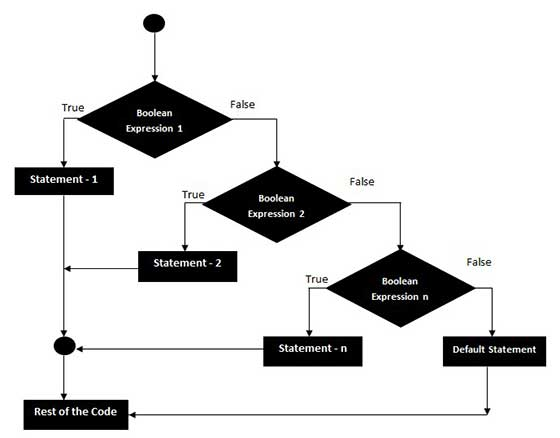# VBA - If Elseif - Else statement

An If statement followed by one or more ElseIf statements that consists of boolean expressions and then followed by a default else statement, which executes when all the condition becomes false.

## Syntax

Following is the syntax of an If Elseif - Else statement in VBScript.

```If(boolean_expression) Then
Statement 1
.....
.....
Statement n
ElseIf (boolean_expression) Then
Statement 1
.....
....
Statement n
ElseIf (boolean_expression) Then
Statement 1
.....
....
Statement n
Else
Statement 1
.....
....
Statement n
End If
```

## Flow Diagram### Example

For demo purpose, let us find the biggest between the two numbers of an Excel with the help of a function.

```Private Sub if_demo_Click()
Dim x As Integer
Dim y As Integer

x = 234
y = 234

If x > y Then
MsgBox "X is Greater than Y"
ElseIf y > x Then
Msgbox "Y is Greater than X"
Else
Msgbox "X and Y are EQUAL"
End If
End Sub
```

When the above code is executed, it produces the following result.

```X and Y are EQUAL
```
vba_decisions.htm